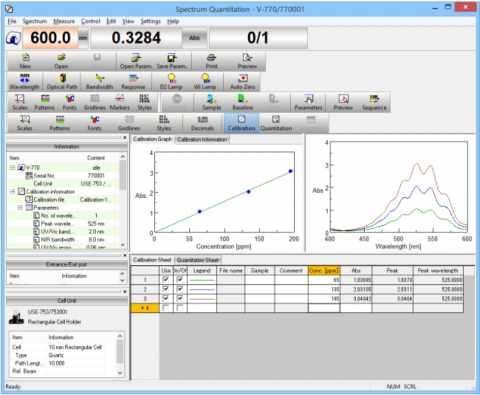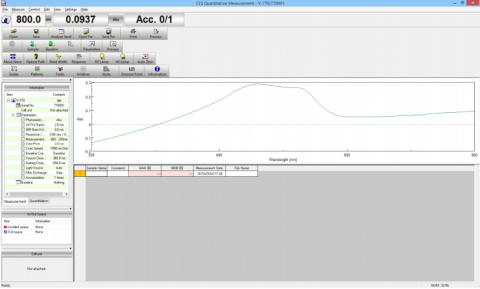# Quantitation and ChemometricsVWQM-978 | Spectrum Quantitative Measurement
Measure the spectrum of a sample and perform quantitation using either the absorbance at a specified wavelength, or the peak absorbance for a standard sample with maximum concentration. The quantitation method can be selected from the following: no base (1-wavelength quantitative analysis), one-point base (2-wavelength quantitative analysis), or two-point base (3-wavelength quantitative analysis).WCL-954 | CLS Quantitative Measurement
Measure samples and automatically perform a quantitative analysis using the classical least squares (CLS) method. A CLS calibration model must first be created and contains correlation curves for quantifying the normal absorbance of the components to be analyzed.

VWPC-955 | PCR Quantitative Measurement
Measure samples and automatically perform a quantitative analysis using the principle component regression (PCR) method. A PCR calibration model must first be created and contains correlation curves for quantifying the normal absorbance of the components to be analyzed.

VWPL-956 | PLS Quantitative Measurement
Measure samples and automatically perform a quantitative analysis using the partial least squares (PLS) method. A PLS calibration model must first be created and contains correlation curves for quantifying the normal absorbance of the components to be analyzed.